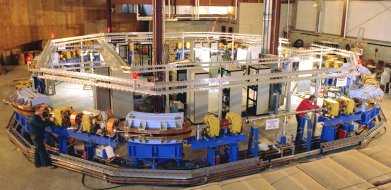# Special relativity / Elementary Tour part 6: E=mc²

Now that the new features of space and time are sufficiently explored, it’s time to examine how this affects the way objects move, either freely or when influenced by forces. In other words: How does relativistic mechanics work? This question has led to the prediction (and, eventually, the measurement) of further relativistic effects, such as the following. In pre-Einstein physics, the ratio of the strength of a force acting on a solid object and the resulting change in the object’s velocity (its acceleration) was thought to be constant. Physicists called it the object’s mass (more precisely: its inertial mass).

In special relativity, however, the inertial mass of a body directly depends on its speed – the higher the speed, the higher the mass. This effect is crucial for anyone operating a high-energy particle accelerator in which elementary particles are accelerated to speeds near that of light. The following image shows one such accelerator, the VUV ring at Brookhaven National Laboratory, with a circumference of 51 metres:[© Courtesy of Brookhaven National Laboratory]

The machine is used to produce what is called synchrotron radiation – a form of electromagnetic radiation that is especially concentrated and intensive, and that can be used for a variety of investigations from basic physics to materials science to medicine. Inside this accelerator, electrons are racing around at slightly more than 99.9999 per cent of the speed of light. If one were to ignore the relativistic mass increase, one couldn’t even get this machine to run.

The relativistic increase of mass happens in a way that makes it impossible to accelerate an object to light speed: The faster the object already is, the more difficult any further acceleration becomes. The closer the object’s speed is to light speed, the greater the increase in inertial mass; to reach light speed exactly would require an infinitely strong force acting on the body. This enforces special relativity’s speed limit: No material object can be accelerated to light speed.

The increase in inertial mass is part of a more general phenomenon, the relativistic equivalence of mass and energy: If one adds energy to a body, one automatically increases its mass; if one takes energy away from it, one decreases its mass. In the case of acceleration, the object in question gains kinetic energy (“movement energy”), and this increase in energy automatically means an increase in mass.

On the other hand, even an object at rest turns out to have a certain amount of energy. Energy and (inertial) mass are inextricably linked by Einstein’s famous formula. Every body of mass m will necessarily have a total energy

E = mc²

(where the constant c is the speed of light). Inverting the formula, every body which has the total energy E will have an inertial mass m = E/c². Although physicists used to measure them in different units (which necessitate the conversion factor c²), mass and energy really are the same. The only reason pre-Einstein physicists defined them as separate concepts in the first place is because they didn’t yet know the whole picture.